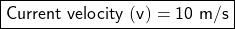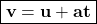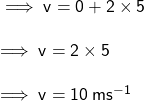## 2. Andy is accelerating at 2 m/s?. If he started from a stand still and he has been accelerating for 5 seconds, what is his current ve

Question

2. Andy is accelerating at 2 m/s?. If he started from a stand still and he has been
accelerating for 5 seconds, what is his current velocity?

in progress 0
7 months 2021-07-27T08:16:12+00:00 1 Answers 4 views 0Given:

Initial velocity (u) = 0 m/s (Started from a stand still)

Acceleration (a) = 2 m/s²

Time taken (t) = 5 seconds

To Find:

Current velocity (v)

Explanation:

From equation of motion we have:By substituting value of u, a & t in the equation we get: